Next: Varying n for Speed Up: Karhunen-Loeve Decomposition for Statistical Previous: Encoding Face Images with

## Decoding a Key into an Image

The encoded faces can be synthesized (or mapped back into image space) from their 60-coefficient key (cj) by summing the mean face and the 60 weighted eigenvectors linearly. This forms an approximation n-tuple,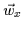, of each original face,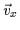, as given by Equation. The remapped approximations for Figureare shown in Figure. Note the low degree of lossiness'' of the compression despite the large compression ratio of 7000 pixels to 60 coefficients (i.e., 117:1 compression).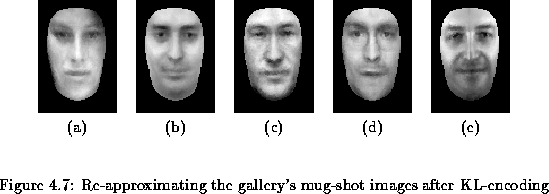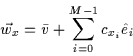(4.36)

We can quantify the lossiness in the KL-based decomposition by measuring the variance between the original vectorand the optimal linear approximation vector. This residual variance, residuex accounts for the total variance in our vector that cannot be spanned by the eigenvectors. The total residual variance in the dataset that cannot be spanned by the eigenvectors is denoted by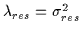and computed using Equation: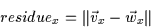(4.37)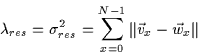(4.38)

We have thus described a method for converting a mug-shot image into a 60-scalar code which describes it in the KL domain. Furthermore, we have shown how this transformation can be inverted so that the 60-element code can be used to regenerate an approximation to the original image. Thus, the KL transform and inverse'' KL transform are added to our palette of tools.Next: Varying n for Speed Up: Karhunen-Loeve Decomposition for Statistical Previous: Encoding Face Images with
Tony Jebara
2000-06-23